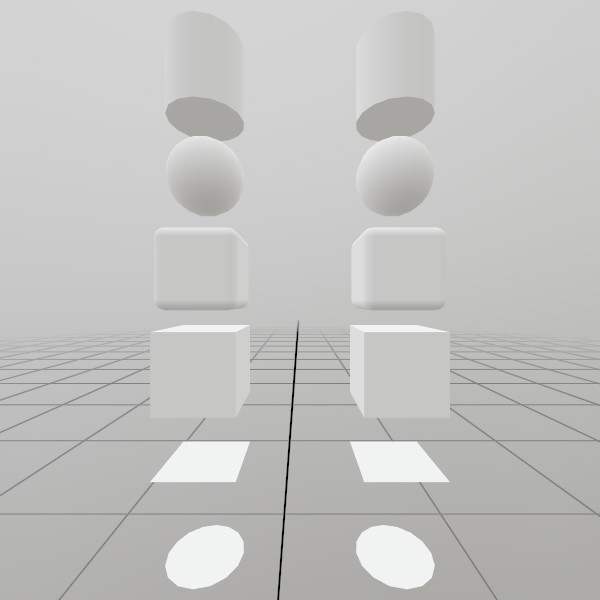# Mesh.Draw

``````void Draw(Material material, Matrix transform, Color colorLinear, RenderLayer layer)
``````

Adds a mesh to the render queue for this frame! If the Hierarchy has a transform on it, that transform is combined with the Matrix provided here.

Material material A Material to apply to the Mesh.
Matrix transform A Matrix that will transform the mesh from Model Space into the current Hierarchy Space.
Color colorLinear A per-instance linear space color value to pass into the shader! Normally this gets used like a material tint. If you’re adventurous and don’t need per-instance colors, this is a great spot to pack in extra per-instance data for the shader!
RenderLayer layer All visuals are rendered using a layer bit-flag. By default, all layers are rendered, but this can be useful for filtering out objects for different rendering purposes! For example: rendering a mesh over the user’s head from a 3rd person perspective, but filtering it out from the 1st person perspective.
``````void Draw(Material material, Matrix transform)
``````

Adds a mesh to the render queue for this frame! If the Hierarchy has a transform on it, that transform is combined with the Matrix provided here.

Material material A Material to apply to the Mesh.
Matrix transform A Matrix that will transform the mesh from Model Space into the current Hierarchy Space.

## Examples

### Generating a Mesh and ModelHere’s a quick example of generating a mesh! You can store it in just a Mesh, or you can attach it to a Model for easier rendering later on.

``````// Do this in your initialization
Mesh  roundedCubeMesh  = Mesh.GenerateRoundedCube(Vec3.One * 0.4f, 0.05f);
Model roundedCubeModel = Model.FromMesh(roundedCubeMesh, Default.Material);
``````

Drawing both a Mesh and a Model generated this way is reasonably simple, here’s a short example! For the Mesh, you’ll need to create your own material, we just loaded up the default Material here.

``````// Call this code every Step

Matrix roundedCubeTransform = Matrix.T(-.5f, 0, 0);
roundedCubeMesh.Draw(Default.Material, roundedCubeTransform);

roundedCubeTransform = Matrix.T(.5f, 0, 0);
roundedCubeModel.Draw(roundedCubeTransform);
``````

Found an issue with these docs, or have some additional questions? Create an Issue on Github!